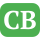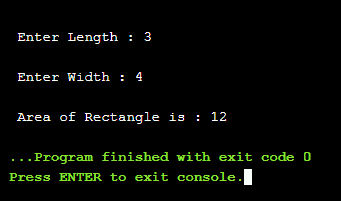CppBuzz.com

# C++ Program to calculate area of Rectangle

```#include<iostream>
using namespace std;

float area(float length, float width){
return (length*width);
}

int main()
{
float length, width, rec_area;

cout<<"\n Enter Length : ";
cin>>length;

cout<<"\n Enter Width : ";
cin>>width;

rec_area = area(length, width);

cout<<"\n Area of Rectangle is : "<< rec_area;

return 0;
}
```

## Area of Rectangle when Length is 3, Width is 4